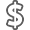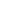# Essentials of Probability & Statistics for Engineers & Scientists

• ISBN13:

• ISBN10:

## 0321783735

• Edition: 1st
• Format: Hardcover
• Publisher: Pearson

Note: Supplemental materials are not guaranteed with Rental or Used book purchases.

Purchase Benefits

•Free Shipping On Orders Over \$35!
Your order must be \$35 or more to qualify for free economy shipping. Bulk sales, PO's, Marketplace items, eBooks and apparel do not qualify for this offer.
•Get Rewarded for Ordering Your Textbooks! Enroll Now
•We Buy This Book Back!
In-Store Credit: \$17.72
Check/Direct Deposit: \$16.88
PayPal: \$16.88
List Price: \$193.32 Save up to \$149.33
• Rent Book \$86.99Free Shipping

TERM
PRICE
DUE
USUALLY SHIPS IN 2-3 BUSINESS DAYS
*This item is part of an exclusive publisher rental program and requires an additional convenience fee. This fee will be reflected in the shopping cart.

### Supplemental Materials

What is included with this book?

• The New copy of this book will include any supplemental materials advertised. Please check the title of the book to determine if it should include any access cards, study guides, lab manuals, CDs, etc.
• The Used, Rental and eBook copies of this book are not guaranteed to include any supplemental materials. Typically, only the book itself is included. This is true even if the title states it includes any access cards, study guides, lab manuals, CDs, etc.

### Summary

This text covers the essential topics needed for a fundamental understanding of basic statistics and its applications in the fields of engineering and the sciences. Interesting, relevant applications use real data from actual studies, showing how the concepts and methods can be used to solve problems in the field. The authors assume one semester of differential and integral calculus as a prerequisite.

1. Introduction to Statistics and Probability

1.1 Overview: Statistical Inference, Samples, Populations, and the Role of Probability

1.2 Sampling Procedures; Collection of Data

1.3 Discrete and Continuous Data.

1.4 Probability: Sample Space and Events

Exercises

1.5 Counting Sample Points

Exercises

1.6 Probability of an Event

Exercises

1.8 Conditional Probability, Independence, and the Product Rule

Exercises

1.9 Bayes' Rule

Exercises

Review Exercises

2. Random Variables, Distributions, and Expectations

2.1 Concept of a Random Variable

2.2 Discrete Probability Distributions

2.3 Continuous Probability Distributions

Exercises

2.4 Joint Probability Distributions

Exercises

2.5 Mean of a Random Variable

Exercises

2.6 Variance and Covariance of Random Variables.

Exercises

2.7 Means and Variances of Linear Combinations of Random Variables

Exercises

Review Exercises

2.8 Potential Misconceptions and Hazards; Relationship to Material in Other Chapters

3. Some Probability Distributions

3.1 Introduction and Motivation

3.2 Binomial and Multinomial Distributions

Exercises

3.3 Hypergeometric Distribution

Exercises

3.4 Negative Binomial and Geometric Distributions

3.5 Poisson Distribution and the Poisson Process

Exercises

3.6 Continuous Uniform Distribution

3.7 Normal Distribution

3.8 Areas under the Normal Curve

3.9 Applications of the Normal Distribution

Exercises

3.10 Normal Approximation to the Binomial

Exercises

3.11 Gamma and Exponential Distributions

3.12 Chi-Squared Distribution.

Exercises

Review Exercises

3.13 Potential Misconceptions and Hazards; Relationship to Material in Other Chapters

4. Sampling Distributions and Data Descriptions

4.1 Random Sampling

4.2 Some Important Statistics

Exercises

4.3 Sampling Distributions

4.4 Sampling Distribution of Means and the Central Limit Theorem

Exercises

4.5 Sampling Distribution of S2

4.6 t-Distribution

4.7 F-Distribution

4.8 Graphical Presentation

Exercises

Review Exercises

4.9 Potential Misconceptions and Hazards; Relationship to Material in Other Chapters

5. One- and Two-Sample Estimation Problems

5.1 Introduction

5.2 Statistical Inference

5.3 Classical Methods of Estimation.

5.4 Single Sample: Estimating the Mean

5.5 Standard Error of a Point Estimate

5.6 Prediction Intervals

5.7 Tolerance Limits

Exercises

5.8 Two Samples: Estimating the Difference between Two Means

5.9 Paired Observations

Exercises

5.10 Single Sample: Estimating a Proportion

5.11 Two Samples: Estimating the Difference between Two Proportions

Exercises

5.12 Single Sample: Estimating the Variance

Exercises

Review Exercises

5.13 Potential Misconceptions and Hazards; Relationship to Material in Other Chapters

6. One- and Two-Sample Tests of Hypotheses.

6.1 Statistical Hypotheses: General Concepts

6.2 Testing a Statistical Hypothesis

6.3 The Use of P-Values for Decision Making in Testing Hypotheses

Exercises

6.4 Single Sample: Tests Concerning a Single Mean

6.5 Two Samples: Tests on Two Means

6.6 Choice of Sample Size for Testing Means

6.7 Graphical Methods for Comparing Means

Exercises

6.8 One Sample: Test on a Single Proportion.

6.9 Two Samples: Tests on Two Proportions

Exercises

6.10 Goodness-of-Fit Test

6.11 Test for Independence (Categorical Data)

6.12 Test for Homogeneity

6.13 Two-Sample Case Study

Exercises

Review Exercises

6.14 Potential Misconceptions and Hazards;

Relationship to Material in Other Chapters

7. Linear Regression

7.1 Introduction to Linear Regression

7.2 The Simple Linear Regression (SLR) Model and the Least Squares Method.

Exercises

7.3 Inferences Concerning the Regression Coefficients.

7.4 Prediction

Exercises

7.5 Analysis-of-Variance Approach

7.6 Test for Linearity of Regression: Data with Repeated Observations

Exercises

7.7 Diagnostic Plots of Residuals: Graphical Detection of Violation of Assumptions

7.8 Correlation

7.9 Simple Linear Regression Case Study.

Exercises

7.10 Multiple Linear Regression and Estimation of the Coefficients

Exercises

7.11 Inferences in Multiple Linear Regression

Exercises

Review Exercises

8. One-Factor Experiments: General

8.1 Analysis-of-Variance Technique and the Strategy of Experimental Design

8.2 One-Way Analysis of Variance (One-Way ANOVA): Completely Randomized Design

8.3 Tests for the Equality of Several Variances

Exercises

8.4 Multiple Comparisons

Exercises

8.5 Concept of Blocks and the Randomized Complete Block Design

Exercises

8.6 Random Effects Models

8.7 Case Study for One-Way Experiment

Exercises

Review Exercises

8.8 Potential Misconceptions and Hazards; Relationship to Material in Other Chapters

9. Factorial Experiments (Two or More Factors)

9.1 Introduction

9.2 Interaction in the Two-Factor Experiment

9.3 Two-Factor Analysis of Variance

Exercises

9.4 Three-Factor Experiments.

Exercises

Review Exercises

9.5 Potential Misconceptions and Hazards; Relationship to Material in Other Chapters